Concepts

Computer Science - Class 11
Chapter 6 Class 11 - Flow of Control

1. Program to sort 3 numbers in descending order using if-else statements

``` a = int(input( "Enter first number: " )) ```

``` b = int(input( "Enter second number: " )) ```

``` c = int(input( "Enter third number: " )) ```

``` if a>b: ```

```     if b>c: ```

```         print( "Numbers in sorted order:" ,a, " " ,b, " " ,c) ```

```     else : ```

```         if c>a: ```

```             print( "Numbers in sorted order:" ,c, " " ,a, " " ,b) ```

``` else : ```

```             print( "Numbers in sorted order:" ,a, " " ,c, " " ,b) ```

``` elif b>a: ```

```     if a>c: ```

```           print( "Numbers in sorted order:" ,b, " " ,a, " " ,c) ```

```     else : ```

```             print( "Numbers in sorted order:" ,b, " " ,c, " " ,a) ```

``` else : ```

```     print( "Numbers in sorted order:" ,c, " " ,b, " " ,a) ```

Output: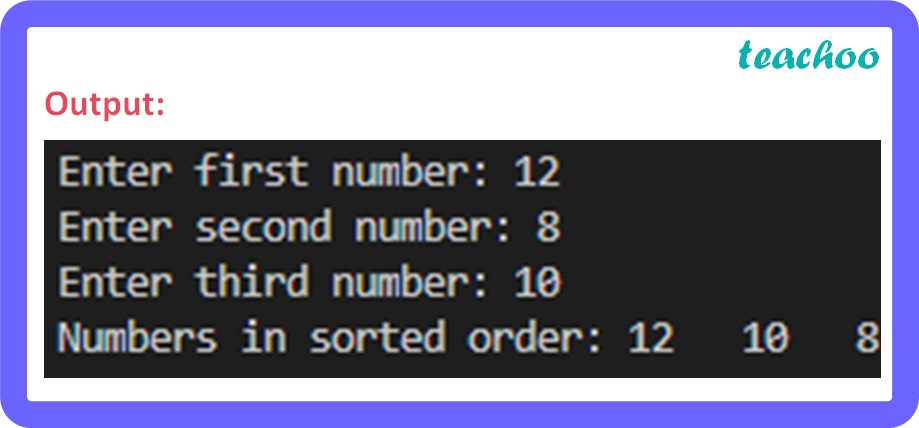2. Program to generate the pattern given below by accepting the input number from user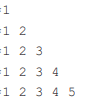``` num = int(input( "Enter a number to generate its pattern = " )) ```

``` for i in range( 1 ,num + 1 ): ```

```     for j in range( 1 ,i + 1 ): ```

```         print(j, end = " " ) ```

```     print() ```

Output: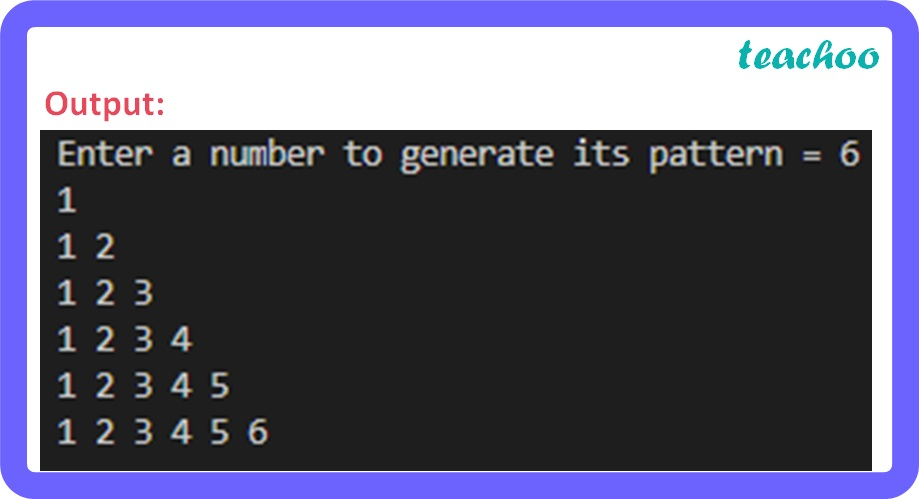3. Program to find sum of series

(1*1) + (2*2) + (3*3) + (4*4) + (5*5) + … + (n*n)

``` num = int(input( "Enter value for n = " )) ```

``` sum= 0 ```

``` for i in range( 1 , num + 1 ): ```

```         sum += (i * i) ```

``` print( "Sum of series = " ,sum) ```

Output: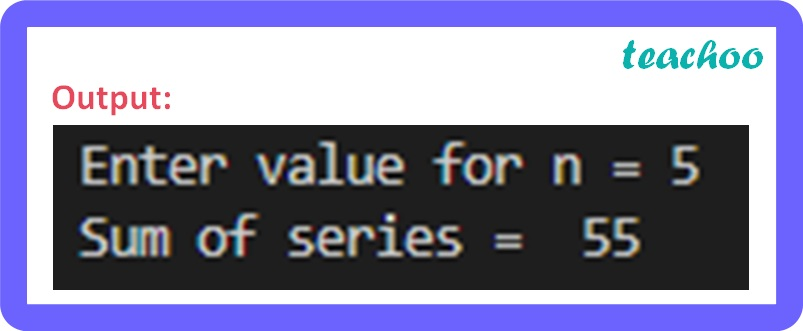4. Program to find factorial of a given number

``` num = int(input( "Enter a number: " )) ```

``` fact = 1 ```

``` if num < 0 : ```

``` print( "Sorry, factorial does not exist for negative numbers" ) ```

``` elif num == 0 : ```

``` print( "The factorial of 0 is 1" ) ```

``` else: ```

```     for i in range( 1 , num + 1 ): ```

```         fact = fact * i ```

``` print("factorial of ", num, " is ", fact) ```

Output: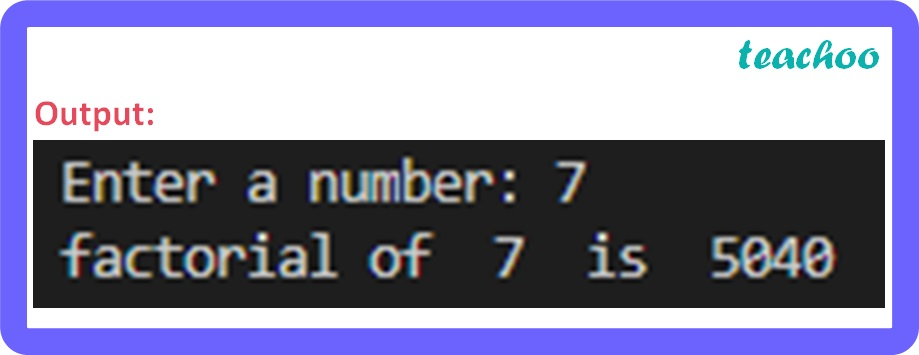Learn in your speed, with individual attention - Teachoo Maths 1-on-1 Class